# RBSE Maths Class 12 Chapter 13: Vector Important Questions and Solutions

RBSE Class 12 Maths Chapter 13 – Vector Important questions and solutions are available here. The important questions and solutions for this chapter of Class 12 are given as per the updated syllabus. The RBSE Class 12 important questions and solutions provided at BYJU’S have covered all the concepts so that students can get a complete grip on the syllabus.

Chapter 13 of RBSE Class 12 has five exercises which contain different types of questions on vectors. Students will be introduced to the various concepts of vectors such as scalar product, vector product, angle between the given vectors, projection of one vector on another and coplanar condition of vectors.

### RBSE Maths Chapter 13: Exercise 13.1 Textbook Important Questions and Solutions

For the important practice questions and solutions of this exercise, students can visit here.

### RBSE Maths Chapter 13: Exercise 13.2 Textbook Important Questions and Solutions

Question 1: If the magnitude of two vectors is 4 and 5, then find their scalar product if the angle between the two vectors is:

(i) 60° (ii) 90°

Solution:

If

$$\begin{array}{l}\vec{a}\end{array}$$
and
$$\begin{array}{l}\vec{b}\end{array}$$
be the two vectors and θ be the angle between them, then
$$\begin{array}{l}cos\theta=\frac{\vec{a}.\vec{b}}{|\vec{a}|.|\vec{b}|}\end{array}$$

(i) Given,

θ = 60°,

$$\begin{array}{l}|\vec{a}|=4,|\vec{b}|=5\end{array}$$

$$\begin{array}{l}cos\ 60^{\circ}=\frac{\vec{a}.\vec{b}}{4.5}\\\frac{1}{2}=\frac{\vec{a}.\vec{b}}{20}\\\vec{a}.\vec{b}=\frac{20}{2}\\\vec{a}.\vec{b}=10\end{array}$$

Therefore, the scalar product of the vectors = 10

(ii) θ = 90°,

$$\begin{array}{l}|\vec{a}|=4,|\vec{b}|=5\end{array}$$

$$\begin{array}{l}cos\ 90^{\circ}=\frac{\vec{a}.\vec{b}}{4.5}\\0=\frac{\vec{a}.\vec{b}}{20}\\\Rightarrow \vec{a}.\vec{b}=0\end{array}$$

Therefore, the scalar product of the vectors = 0

Question 2: Find the value of

$$\begin{array}{l}\vec{a}.\vec{b}\end{array}$$
if
$$\begin{array}{l}\vec{a}=2\hat{i}+5\hat{j}, \vec{b}=3\hat{i}-2\hat{j}.\end{array}$$

Solution:

Given,

$$\begin{array}{l}\vec{a}=2\hat{i}+5\hat{j}, \vec{b}=3\hat{i}-2\hat{j}.\end{array}$$

This can be written as

$$\begin{array}{l}\vec{a}=2\hat{i}+5\hat{j}+0\hat{k}, \vec{b}=3\hat{i}-2\hat{j}+0\hat{k}.\end{array}$$

$$\begin{array}{l}\vec{a}.\vec{b}=(2\hat{i}+5\hat{j}+0\hat{k}).(3\hat{i}-2\hat{j}+0\hat{k})\end{array}$$

= (2 × 3) + [5 × (-2)] + (0 × 0)

= 6 + (-10) + 0

= 6 – 10

= -4

Question 3: For any vector

$$\begin{array}{l}\vec{a}\end{array}$$
, prove that
$$\begin{array}{l}\vec{a}=(\vec{a}.\hat{i})\hat{i}+(\vec{a}.\hat{j})\hat{j}+(\vec{a}.\hat{k})\hat{k}\end{array}$$

Solution:

Let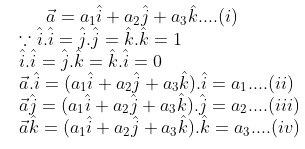Substituting (ii), (iii), and (iv) in (i),

$$\begin{array}{l}\vec{a}=(\vec{a}.\hat{i})\hat{i}+(\vec{a}.\hat{j})\hat{j}+(\vec{a}.\hat{k})\hat{k}\end{array}$$

### RBSE Maths Chapter 13: Exercise 13.3 Textbook Important Questions and Solutions

Question 4: Find the vector product of

$$\begin{array}{l}3\hat{i}+\hat{j}-\hat{k}\end{array}$$
and
$$\begin{array}{l}2\hat{i}+3\hat{j}+\hat{k}\end{array}$$
.

Solution:

Let

$$\begin{array}{l}\vec{a}=3\hat{i}+\hat{j}-\hat{k}\\\vec{b}=2\hat{i}+3\hat{j}+\hat{k}\end{array}$$

The cross product of given vectors is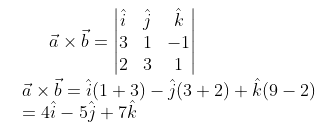Question 5: Find the vector with magnitude 9 units which is perpendicular to the vectors

$$\begin{array}{l}4\hat{i}-\hat{j}+3\hat{k}\end{array}$$
and
$$\begin{array}{l}-2\hat{i}+\hat{j}-2\hat{k}\end{array}$$

Solution:

Let

$$\begin{array}{l}\vec{a}=4\hat{i}-\hat{j}+3\hat{k}\end{array}$$
and
$$\begin{array}{l}\vec{b}=-2\hat{i}+\hat{j}-2\hat{k}\end{array}$$

Now,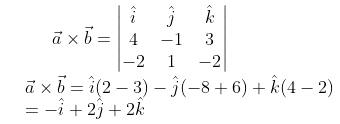Perpendicular unit vector along

$$\begin{array}{l}\vec{a}\times \vec{b}\end{array}$$
is: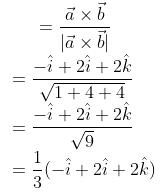### RBSE Maths Chapter 13: Exercise 13.4 Textbook Important Questions and Solutions

Question 6: If

$$\begin{array}{l}\vec{a}=2\hat{i}-3\hat{j}+4\hat{k},\vec{b}=\hat{i}+2\hat{j}-\hat{k}\end{array}$$

and

$$\begin{array}{l}\vec{c}=3\hat{i}-\hat{j}+2\hat{k}\end{array}$$
, then find
$$\begin{array}{l}[\vec{a}\vec{b}\vec{c}]\end{array}$$
.

Solution:

Given,

$$\begin{array}{l}\vec{a}=2\hat{i}-3\hat{j}+4\hat{k},\vec{b}=\hat{i}+2\hat{j}-\hat{k}\end{array}$$

and

$$\begin{array}{l}\vec{c}=3\hat{i}-\hat{j}+2\hat{k}\end{array}$$

$$\begin{array}{l}[\vec{a}\vec{b}\vec{c}]=\begin{vmatrix} 2 & -3 & 4\\ 1 & 2 & -1\\ 3 & -1 & 2 \end{vmatrix}\end{array}$$

= 2(4 -1) + 3(2 + 3) + 4(-1 – 6)

= 2(3) + 3(5) + 4(-7)

= 6 + 15 – 28

= -7

Question 7: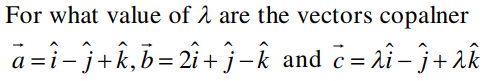Solution:

Let the given vectors be: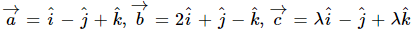Given that, the vectors are coplanar.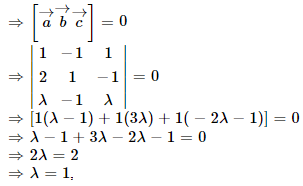### RBSE Maths Chapter 13: Exercise 13.5 Textbook Important Questions and Solutions

Question 8: Prove that: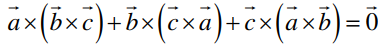Solution: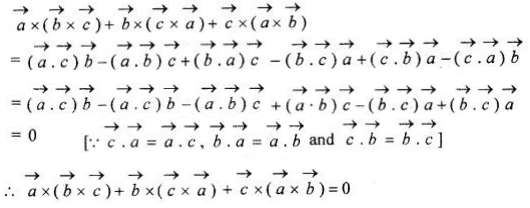Hence proved.

Question 9: Find the projection vector

$$\begin{array}{l}\hat{i}+\hat{j}\end{array}$$
on
$$\begin{array}{l}\hat{i}-\hat{j}\end{array}$$

Solution:

Let

$$\begin{array}{l}\vec{a}=\hat{i}+\hat{j}\end{array}$$

$$\begin{array}{l}\vec{b}=\hat{i}-\hat{j}\end{array}$$

We know that the projection of

$$\begin{array}{l}\vec{a}\end{array}$$
on
$$\begin{array}{l}\vec{b}\end{array}$$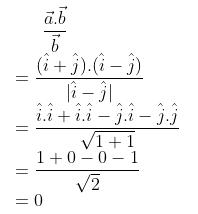Therefore, the projection vector

$$\begin{array}{l}\hat{i}+\hat{j}\end{array}$$
on
$$\begin{array}{l}\hat{i}-\hat{j}\end{array}$$
is 0.